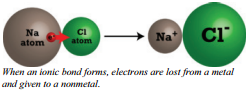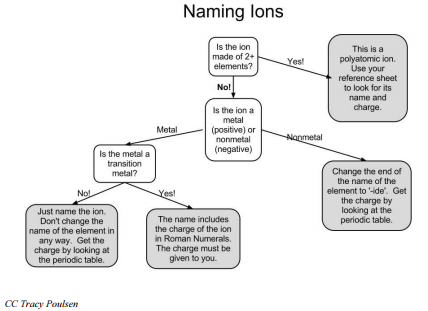# 4.3: Names and Charges of Ions

$$\newcommand{\vecs}{\overset { \rightharpoonup} {\mathbf{#1}} }$$ $$\newcommand{\vecd}{\overset{-\!-\!\rightharpoonup}{\vphantom{a}\smash {#1}}}$$$$\newcommand{\id}{\mathrm{id}}$$ $$\newcommand{\Span}{\mathrm{span}}$$ $$\newcommand{\kernel}{\mathrm{null}\,}$$ $$\newcommand{\range}{\mathrm{range}\,}$$ $$\newcommand{\RealPart}{\mathrm{Re}}$$ $$\newcommand{\ImaginaryPart}{\mathrm{Im}}$$ $$\newcommand{\Argument}{\mathrm{Arg}}$$ $$\newcommand{\norm}{\| #1 \|}$$ $$\newcommand{\inner}{\langle #1, #2 \rangle}$$ $$\newcommand{\Span}{\mathrm{span}}$$ $$\newcommand{\id}{\mathrm{id}}$$ $$\newcommand{\Span}{\mathrm{span}}$$ $$\newcommand{\kernel}{\mathrm{null}\,}$$ $$\newcommand{\range}{\mathrm{range}\,}$$ $$\newcommand{\RealPart}{\mathrm{Re}}$$ $$\newcommand{\ImaginaryPart}{\mathrm{Im}}$$ $$\newcommand{\Argument}{\mathrm{Arg}}$$ $$\newcommand{\norm}{\| #1 \|}$$ $$\newcommand{\inner}{\langle #1, #2 \rangle}$$ $$\newcommand{\Span}{\mathrm{span}}$$

Skills to Develop

• Describe how atoms form an ionic bond.
• Indicate the most likely number of electrons the atom will gain or lose when forming an ion.
• Describe what polyatomic ions are.​

Molecular collisions between atoms that tend to lose electrons (metals) and atoms that tend to gain electrons (nonmetals) are sometimes sufficient to remove electrons from the metal atoms and add them to the nonmetal atom. This transference of electrons from metals to nonmetals forms positive and negative ions, which in turn, attract each other due to opposite charges. The compounds formed by this electrostatic attraction are said to be ionically bonded.

## Ionic BondingThe process of transferring an electron from a sodium atom to a chlorine atom as shown in the diagram produces oppositely charged ions which then stick together because of electrostatic attraction. Electrostatic attraction is the attraction between opposite charges. The electrostatic attraction between oppositelycharged ions is called an ionic bond. These ions are chemically more stable than the atoms were.

If we had been using sodium and sulfur atoms for the transfer discussion, the process would be only slightly different. Sodium atoms have a single electron in their outermost energy level and therefore can lose only one electron. Sulfur atoms, however, require two electrons to complete their outer energy level. In such a case, two sodium atoms would be required to collide with one sulfur atom. Each sodium atom would contribute one electron for a total of two electrons and the sulfur atom would take on both electrons. The two $$\ce{Na}$$ atoms would become $$\ce{Na^+}$$ ions and the sulfur atom would become a $$\ce{S^{2-}}$$ ion. Electrostatic attraction would cause all three ions to stick together.

The cation forming elements, metals, lose all valence electrons so the electron configuration for the ions formed will have the eight electrons of the previous noble gas. (Those whose electron dot formula matches helium, of course, will have only two.) The anion forming elements, nonmetals, will gain enough electrons so the electron dot formulas of their ions will match those of the following noble gas. In all cases, for the "A" group elements, the ions will have eight electrons in their electron dot formula. The octet rule is an expression of this end result of eight electrons in the outermost energy level.

An atom becomes an ion when it gains or loses electrons. The ions that are formed when an atom loses electrons are positively charged because they have more protons in the nucleus than electrons in the electron cloud. Positively charged ions are called cations (pronounced CAT-ions). The ions that are formed when an atom gains electrons are negatively charged because they have more electrons in the electron cloud than protons in the nucleus. Negatively charged ions are called anions (pronounced AN-ions).

## Predicting Charges of Main Group Ions

All the metals in family 1A have electron configurations ending with an $$s^1$$ electron in the outer energy level. For that reason, all family 1A members will tend to lose exactly one electron when they are ionized, obtaining an electron configuration like the closest noble gas. The entire family forms $$+1$$ ions: $$\ce{Li^+}$$, $$\ce{Na^+}$$, $$\ce{K^+}$$, etc. We need to note that while hydrogen is in this same column, it is not considered to be a metal. There are times that hydrogen acts as if it is a metal and forms $$+1$$ ions; however, most of the time it bonds with other atoms as a nonmetal. In other words, hydrogen doesn't easily fit into any chemical family. All members of family 1A form ions with $$+1$$ charge.

The metals in family 2A all have electron configurations ending with two electrons in an $$s^2$$ position in the outermost energy level. To have an electron configuration like the closest noble gas, each of the elements in this family will lose two valence electrons and form $$+2$$ ions; $$\ce{Be^{2+}}$$, $$\ce{Mg^{2+}}$$, etc. Other metal elements' charges can be predicted using the same patterns. Members of family 3A form ions with $$3+$$ charge.

Family 5A nonmetals at the top will gain electrons to form negative ions. By gaining three electrons, they are able to obtain the electron configuration of the noble gas closest to them on the periodic table. Family 6A nonmetals will gain two electrons to obtain an octet thus forming a $$2-$$ ion. Family 7A will form $$1-$$ ions: $$\ce{F^-}$$, $$\ce{Cl^-}$$, etc.

Family 8A, of course, is the noble gases and has no tendency to either gain or lose electrons so they do not form ions.

The charges ions form can be summarized as in the following table. Many of the transition elements have variable oxidation states so they can form ions with different charges, and therefore, are left off this chart.When main group nonmetals gain electrons to form anions, their names are changed to end in "-ide". For example, fluorine atoms gain electrons to become fluoride ions.

## Polyatomic Ions

Thus far, we have been dealing with ions made from single atoms. Such ions are called monatomic ions. There also exists a group of polyatomic ions, ions composed of a group of atoms that are covalently bonded and behave as if they were a single ion. Almost all of the common polyatomic ions are negative ions.

A table of many common polyatomic ions is given. The more familiar you become with polyatomic ions, the better you will be able to write names and formulas of ionic compounds. It is also important to note that there are many polyatomic ions that are not on this chart.## Naming Transition Metals

Some metals are capable of forming ions with various charges. These include most of the transition metals and many post-transition metals. Iron, for example, may form $$\ce{Fe^{2+}}$$ ions by losing 2 electrons or $$\ce{Fe^{3+}}$$ ions by losing 3 electrons. The rule for naming these ions is to insert the charge (oxidation number) of the ion with Roman numerals in parentheses after the name. These two ions would be named iron (II) and iron (III). When you see that the compound involves any of the variable oxidation number metals (iron, copper, tin, lead, nickel, and gold), you must determine the charge (oxidation number) of the metal from the formula and insert Roman numerals indicating that charge.

Consider $$\ce{FeO}$$ and $$\ce{Fe_2O_3}$$. These are very different compounds with different properties. When we name these compounds, it is absolutely vital that we clearly distinguish between them. They are both iron oxides but in $$\ce{FeO}$$ iron is exhibiting a charge of $$2+$$ and in $$\ce{Fe_2O_3}$$, it is exhibiting a charge of $$3+$$. The first, $$\ce{FeO}$$, is named iron (II) oxide. The second, $$\ce{Fe_2O_3}$$, is named iron (III) oxide.

## Lesson Summary

• When an atom gains one or more extra electrons, it becomes a negative ion, an anion.
• When an atom loses one or more of its electrons, it becomes a positive ion, a cation.
• Polyatomic ions are ions composed of a group of atoms that are covalently bonded and behave as if they were a single ion.
• Some transition elements have fixed oxidation numbers and some have variable charges. When naming these charge-variable ions, their charges are included in Roman numerals.## Vocabulary

• Ion: An atom or group of atoms with an excess positive or negative charge.
• Cation: A positive ion.
• Anion: A negative ion.
• Ionic bond: A bond between ions resulting from the transfer of electrons from one of the bonding atoms to the other and the resulting electrostatic attraction between the ions.
• Electrostatic attraction: The force of attraction between opposite electric charges.
• Octet rule: The tendency for atoms to gain or lose the appropriate number of electrons so that the resulting ion has either completely filled or completely empty outer energy levels, or 8 valence electrons.